Courses

# Test: Centre of Mass- 2

## 25 Questions MCQ Test Physics For JEE | Test: Centre of Mass- 2

Description
This mock test of Test: Centre of Mass- 2 for Class 11 helps you for every Class 11 entrance exam. This contains 25 Multiple Choice Questions for Class 11 Test: Centre of Mass- 2 (mcq) to study with solutions a complete question bank. The solved questions answers in this Test: Centre of Mass- 2 quiz give you a good mix of easy questions and tough questions. Class 11 students definitely take this Test: Centre of Mass- 2 exercise for a better result in the exam. You can find other Test: Centre of Mass- 2 extra questions, long questions & short questions for Class 11 on EduRev as well by searching above.
*Multiple options can be correct
QUESTION: 1

### A body has its centre of mass at the origin. The x-coordinates of the particles

Solution:

The y coordinates of the particles of the body may be all non zero and positive for some cases and negative in other cases.

QUESTION: 2

### An object comprises of a uniform ring of radius R and its uniform chord AB (not necessarily made of the same material) as shown. Which of the following can not be the centre of mass of the object.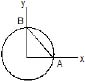Solution: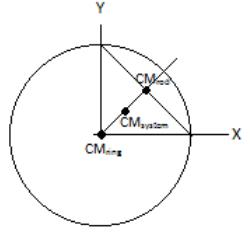Since the points must have symmetric coordinates so B is the answer.

*Multiple options can be correct
QUESTION: 3

### In which of the following cases the centre of mass of a an rod is certainly not at its centre ?

Solution:
1. It can’t be since the integral would yield a value much greater than R/2
2. It can’t be since the integral would yield a value much less than R/2
3. Assuming the rate of increase and decrease is same the COM could lie in the centre
4. Assuming the rate of increase and decrease is same the COM could lie in the centre

*Multiple options can be correct
QUESTION: 4

Consider following statements

 CM of a uniform semicircular disc of radius R = 2R/p from the centre

 CM of a uniform semicircular ring of radius R = 4R/3p from the centre

 CM of a solid hemisphere of radius R = 4R/3p from the centre

 CM of a hemisphere shell of radius R = R/2 from the centre

Which statements are correct?

Solution:
*Multiple options can be correct
QUESTION: 5

Two blocks A (5kg) and B(2kg) attached to the ends of a spring constant 1120 N/m are placed on a smooth horizontal plane with the spring undeformed. Simultaneously velocities of 3m/s and 10m/s along the line of the spring in the same direction are imparted to A and B then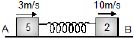Solution:

Vcm = m1v1 + m2v2/m1 + m2 = 5m/s
So, Vrela = -2m/s and Vrelb = 5m/s
Now, Va = 5m/s and Vb = 5m/s
Also, ½ K x2max = ½ μ V2rel
So, xmax = ¼ m = 25 cm
Now, since the time periods of both the blocks are same so they will be at max compression and elongation alternatively

*Multiple options can be correct
QUESTION: 6

Two identical balls are interconnected with a massless and inextensible thread. The system is in gravity free space with the thread just taut. Each ball is imparted a velocity v, one towards the other ball and the other perpendicular to the first, at t = 0. Then,

Solution:
*Multiple options can be correct
QUESTION: 7

A ball moving with a velocity v hits a massive wall moving towards the ball with a velocity u. An elastic impact lasts for a time Dt.

Solution: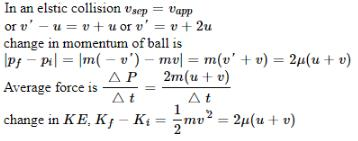*Multiple options can be correct
QUESTION: 8

A particle moving with kinetic energy = 3 joule makes an elastic head on collision with a stationary particle when has twice its mass during the impact.

Solution:
*Multiple options can be correct
QUESTION: 9

Two balls A and B having masses 1 kg and 2 kg, moving with speeds 21 m/s and 4 m/s respectively in opposite direction, collide head on. After collision A moves with a speed of 1 m/s in the same direction, then correct statements is :

Solution:
*Multiple options can be correct
QUESTION: 10

The diagram to the right shown the velocity-time graph for two masses R and S that collided elastically. Which of the following statements is true?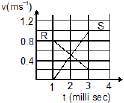(I) R and S moved in the same direction after the collision.

(II) Kinetic energy of the system (R & S) is minimum at t = 2 milli sec.

(III) The mass of R was greater than mass of S.

Solution:
1. The velocities of both the masses are always positive hence same direction
2. The KE will be min when Vr = Vs which is at 2ms
3. The dpr/dt = dps/dt and as we can see in the graph dvr/dt < dvs/dt, so mass of R is greater
*Multiple options can be correct
QUESTION: 11

In an inelastic collision,

Solution:
*Multiple options can be correct
QUESTION: 12

In a one-dimensional collision between two particles, their relative velocity is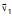before the collision and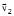after the collision

Solution:
*Multiple options can be correct
QUESTION: 13

A set of n-identical cubical blocks lie at rest parallel to each other along a line on a smooth horizontal surface. The separation between the near surfaces of any two adjacent blocks is L. The block at one end is given a speed V towards the next one at time t = 0. All collision are completely inelastic, then

Solution:
QUESTION: 14

An isolated rail car original moving with speed v0 on a straight, frictionless, level track contains a large amount of sand. A release value on the bottom of the car malfunctions, and sand begins to pour out straight down relative to the rail car.

(a) Is momentum conserved in this process?

(A) The momentum of the rail car along is conserved

(B) The momentum of the rail car + sand remaining within the car is conserved

(C) The momentum of the rail car + all of the sand, both inside and outside the rail car, is conserved

(D) None of the three previous systems have momentum conservation

(b) What happens to the speed of the rail car as the sand pours out?

(A) The car begins to roll faster

(B) The car maintains the same speed

(C) The car begins to slow down

(D) The problem cannot be solved since momentum is not conserved

Solution:
*Multiple options can be correct
QUESTION: 15

If the external forces acting on a system have zero resultant, the centre of mass

Solution:

If the external force acting on a system have zero resultant forces, then the total momentum remains constant i.e. conserved. Hence, the center of mass remains at rest or moves with a constant velocity.

*Multiple options can be correct
QUESTION: 16

A particle of mass m moving horizontal with v0 strikes a smooth wedge of mass M, as shown in figure. After collision, the ball starts moving up the inclined face of the wedge and rises to a height h.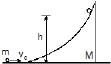The final velocity of the wedge v2 is

Solution:
*Multiple options can be correct
QUESTION: 17

When the particle has risen to a height h on the wedge, then choose the correct alternative(s)

Solution:
*Multiple options can be correct
QUESTION: 18

The maximum height h attained by the particle is

Solution:
*Multiple options can be correct
QUESTION: 19

Identify the correct statement(s) related to the situation when the particle starts moving downward.

Solution:
*Multiple options can be correct
QUESTION: 20

Suppose the particle when reaches the horizontal surfaces, its velocity with respect to ground is v1 and that of wedge is v2. Choose the correct statement (s)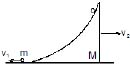Solution:
*Multiple options can be correct
QUESTION: 21

Choose the correct statement(s) related to particle m

Solution:
*Multiple options can be correct
QUESTION: 22

Choose the correct statement related to the wedge M

Solution:
*Multiple options can be correct
QUESTION: 23

A small ball B of mass m is suspended with light inelastic string of length L from a block A of same mass m which can move on smooth horizontal surface as shown in the figure. The ball is displaced by angle q from equilibrium position & then released.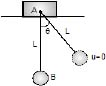The displacement of block when ball reaches the equilibrium position is

Solution:
*Multiple options can be correct
QUESTION: 24

Tension in string when it is vertical, is

Solution:

Tension in string when it is vertical, is Mg. Since nothing else has been provided so we assume all the forces are in the desired directions.

*Multiple options can be correct
QUESTION: 25

Maximum velocity of block during subsequent motion of the system after release of ball is

Solution:

Track your progress, build streaks, highlight & save important lessons and more!

### Similar Content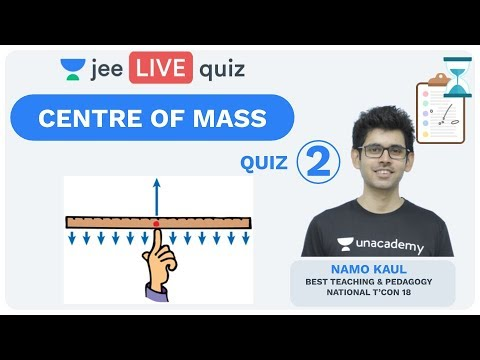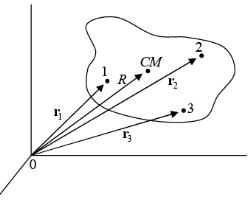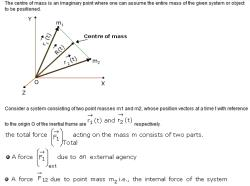### Related tests